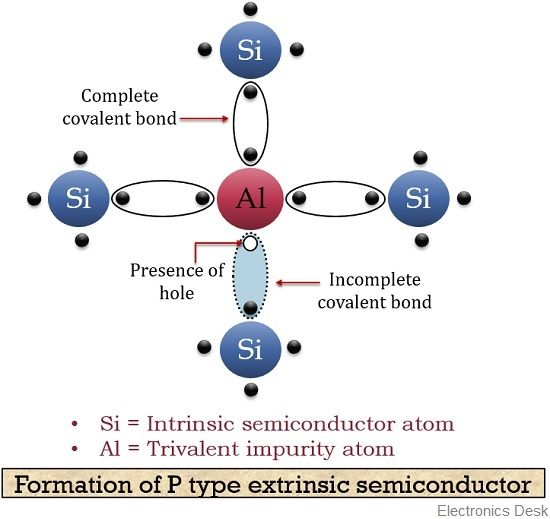# Difference Between P-Type and N-Type Semiconductor

Both p-type and n-type semiconductor is the classification of extrinsic semiconductors. The major factor that generates a difference between p-type and n-type semiconductor lies in their doping material. A p-type semiconductor material is formed by the addition of group III elements or we can say trivalent impurity to a pure (intrinsic) semiconductor. On the contrary, the n-type semiconductor material is formed by the addition of group V elements i.e., a pentavalent impurity to a pure or intrinsic semiconductor.

Some other factors also exist that generates major differences between the two. We will discuss these differences in detail but before proceeding towards that have a look at the contents that are to be discussed under this article.

## Comparison Chart

ParameterP-TypeN-Type
Impurity dopedTrivalent impurityPentavalent impurity
Also known asAcceptor atom because of presence of additional hole.Donor atom due to the existence of additional electron.
Doped groupGroup III elements. For eg - boron, gallium, indium, aluminium etc.Group V elements. for eg - arsenic, antimony, bismuth, phosphorus etc.
Majority carriersHolesElectrons
Minority carriersElectronsHoles
ConductivityDue to presence of holes.Due to presence of electrons.
Presence of fermi levelFermi level appears closer to the valence than the conduction band.Fermi level is present nearer to the conduction band than the valence band.
Concentration of electronsLowVery high as compared to p type semiconductor
Concentration of holesHighComparatively less than p type semiconductor.

### Definition of P-type semiconductor

When a pure or intrinsic semiconductor is doped with group III elements of periodic table like boron, gallium, aluminium etc. that these are known as the p-type extrinsic semiconductor. These are called so because doping these elements will lead to the presence of an extra hole in the valence shell of the atom.

Let us have a look at the figure shown below that represents doping of aluminium to a pure silicon material:As we know that an aluminium atom contains 3 electrons at its valence shell. Also, silicon contains total of 4 electrons at its valence shell. So, 3 valence electrons of aluminium atom make covalent bonds with 3 electrons of silicon. However, in this case, a vacancy of an electron (or a hole) appears. The movement of this hole is mainly responsible for the conduction in the p-type semiconductor to take place. Hence, in this case, charge carriers are holes rather than electrons.

Let us now have a look at the energy level diagram of a p-type semiconductor:Here, we can see that the Fermi level is present near the valence band. As it is clear from the above figure that there exists a very small energy difference between valence band and the acceptor energy level. Thus, electrons easily drift to acceptor energy level creating a vacancy of electrons. Hence, producing holes in the valence band.

### Definition of N-type semiconductor

N-type extrinsic semiconductors are formed when group V elements like phosphorus, antimony, bismuth etc. are doped to a pure semiconductor crystal. These are called so because doping these elements will cause the presence of an additional electron in the valence shell of the atom.

The figure below represents the doping of phosphorus element into a pure silicon material:We already know that phosphorus consists of 5 electrons at its valence shell. When it is doped with pure silicon having 4 electrons at the valence shell then it forms 4 covalent bonds. This leads to the presence of an unbounded electron that is held free to move into the conduction band. This electron is known as a free electron and its movement raises the conductivity of the material.

Now, have a look at the energy band diagram of N-type semiconductor:Here, from the figure, it is clear that the existence of the Fermi level is near the conduction band. We can see that the small energy difference exists between donor energy level and the conduction band. So, less energy is needed by the electrons to reach the conduction band.

## Key Differences between P-Type and N-Type Semiconductor

1. A p-type semiconductor is formed when group III elements are doped to a pure semiconductor material. As against, an n-type semiconductor is formed when group V elements are doped to an intrinsic semiconductor.
2. As elements like boron, gallium, indium etc. are doped to form p-type semiconductor thus it produces an additional hole hence also known as acceptor atom.
On the contrary, elements like arsenic, antimony, bismuth etc. are doped in order to have n-type semiconductor hence it produces an additional electron thus also termed as donor atom.
3. Another factor that generates a key difference between p-type and n-type semiconductor is that in case of p-type semiconductor holes are the majority carriers. While in case of n-type semiconductor electrons act as majority carriers.
4. The minority carriers in case of the p-type semiconductor are electrons whereas in that of n-type semiconductor these are holes.
5. As we know that the conductivity of the device depends on the majority carriers. Thus in the p-type semiconductor, holes are responsible for current conduction. On the contrary, in case of an n-type semiconductor, electrons are responsible for current conduction.
6. The fermi-level in case of p-type semiconductor appears closer to valence band than that of the conduction band. As against, the Fermi level in case of n-type semiconductor exists near the conduction band.
7. The concentration of holes is more than electrons in case of p-type semiconductor material. While in n-type semiconductors the concentration of electrons is greater than holes.

### Conclusion

So, from the above discussion, we can conclude that a p-type semiconductor is abbreviated so because these are positive semiconductors due to presence of extra holes. Whereas, n-type semiconductors are termed as negative semiconductors because of the presence of extra electrons.